# Functional Groups QUEST Organic Chemistry Functional Groups Alkanes

• Slides: 33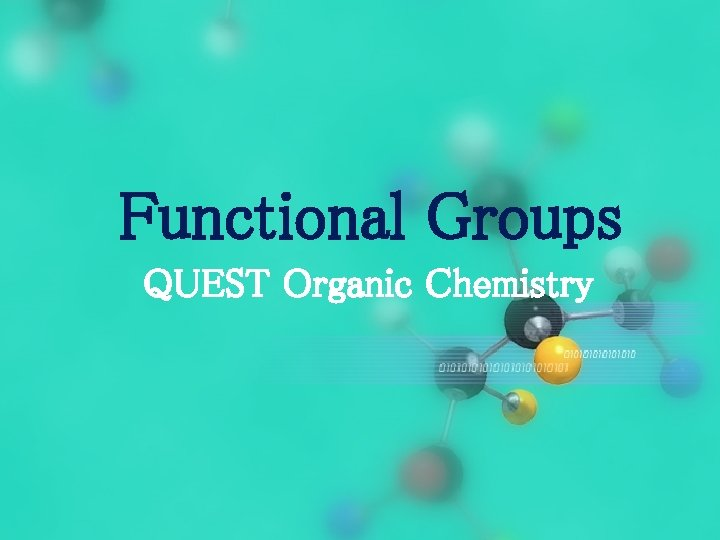Functional Groups QUEST Organic Chemistry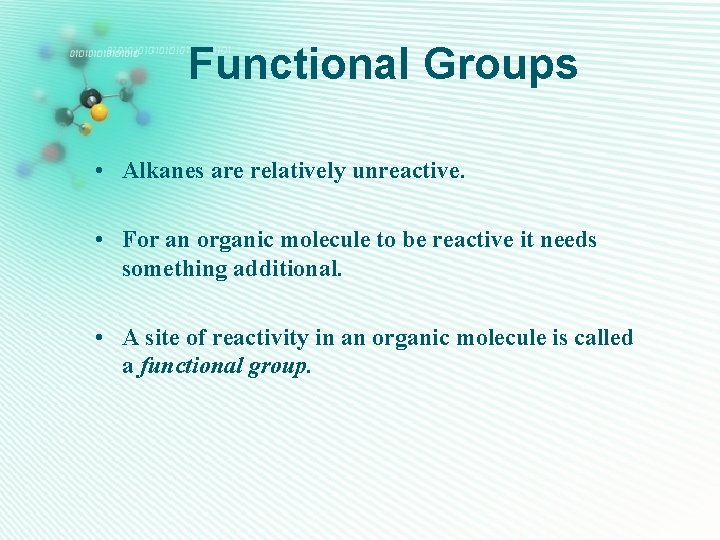Functional Groups • Alkanes are relatively unreactive. • For an organic molecule to be reactive it needs something additional. • A site of reactivity in an organic molecule is called a functional group.Functional Group • A functional group could be an atom or a group of atoms within a molecule that is responsible for its characteristic chemical reactions. • C=C double bond is a functional group. • Other functional groups contain elements other than C or H, notably O, N, S and Cl.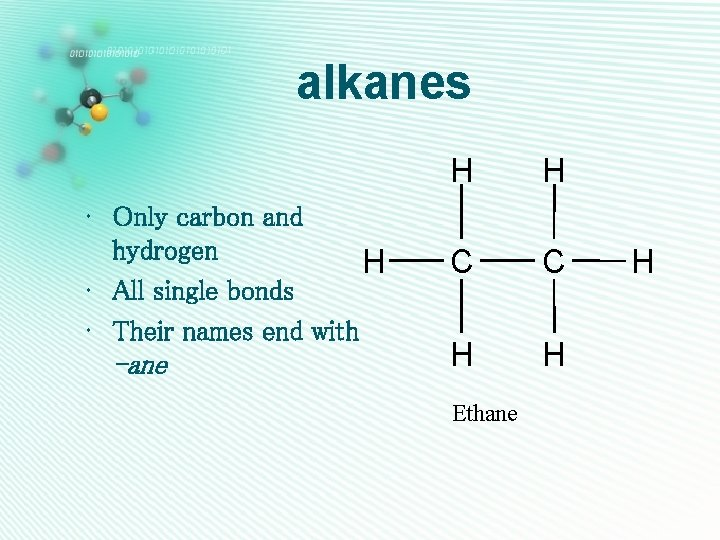alkanes • Only carbon and hydrogen H • All single bonds • Their names end with -ane H H C C H H Ethane H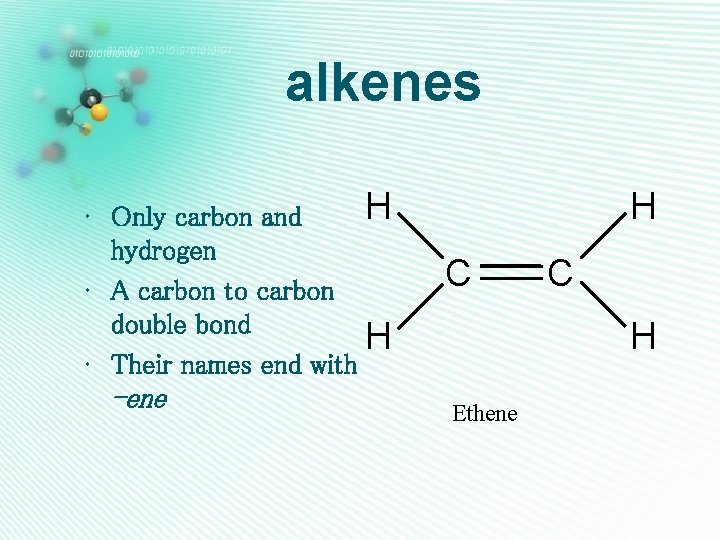alkenes H • Only carbon and hydrogen • A carbon to carbon double bond H • Their names end with -ene H C C H Ethenealkynes • Only carbon and hydrogen • A carbon to carbon triple bond • Their names end with -yne H C Ethyne C Halcohols • Only one oxygen • Has an O-H group H • Can be classified as 1º/2º/3º according to position of O-H group on carbon skeleton • They are named as alkanol H H C C H H Ethanol O H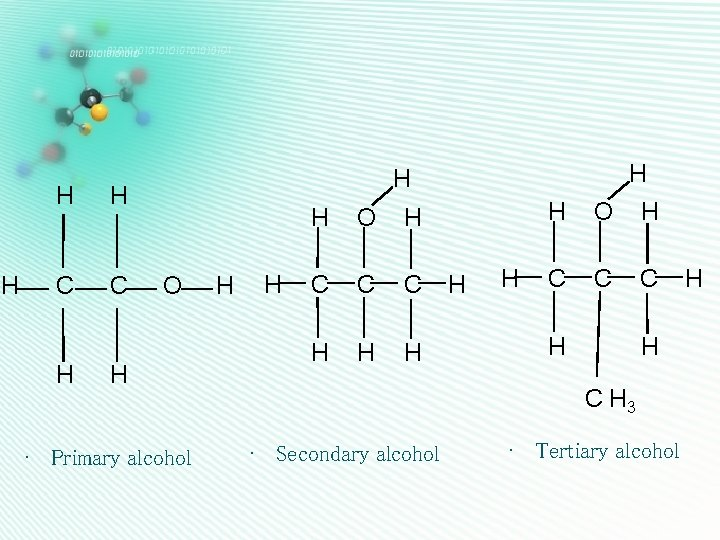H H H C C H H H O H • Primary alcohol H H H O H C C C H H C H 3 • Secondary alcohol • Tertiary alcohol Haldehydes H • Only one oxygen • Has a C=O group C • C=O group is at the end H of the carbon chain, so is next door to a hydrogen H atom Ethanal • They are named as alkanal O C Hketones • Only one oxygen • Has a C=O group • C=O group is not at H the end of carbon chain, so is next door to 2 carbons • They are named as alkanone H O H C C C H H Propanone H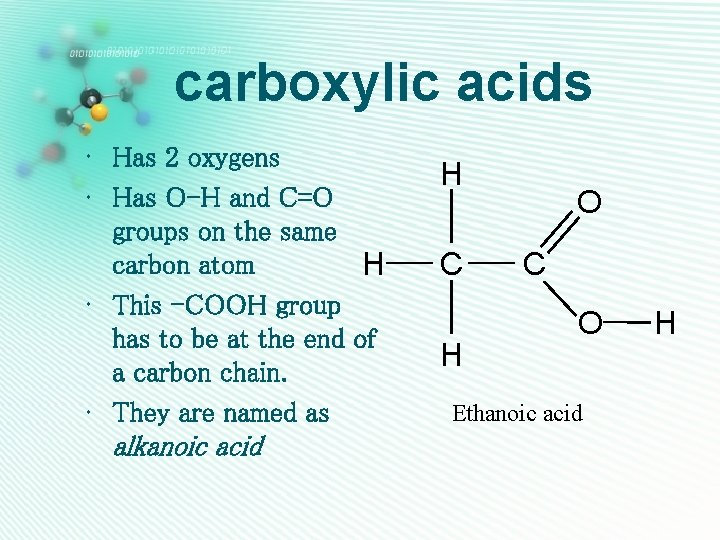carboxylic acids • Has 2 oxygens • Has O-H and C=O groups on the same carbon atom H • This -COOH group has to be at the end of a carbon chain. • They are named as alkanoic acid H C H O C O Ethanoic acid H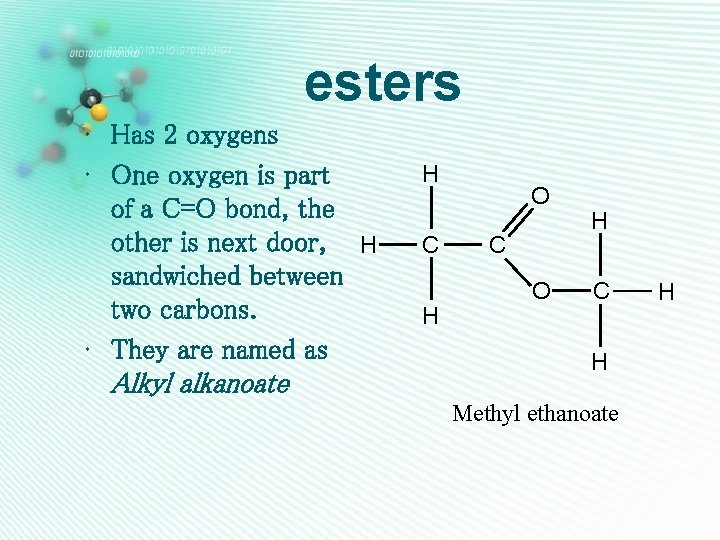esters • Has 2 oxygens • One oxygen is part of a C=O bond, the other is next door, H sandwiched between two carbons. • They are named as Alkyl alkanoate H C H O C O H C H Methyl ethanoate Hether • Has 1 oxygen • No O-H or C=O group H • The oxygen is sandwiched between two carbon atoms • Their names end with -ether H H C C H H H O C H Ethyl methyl ether H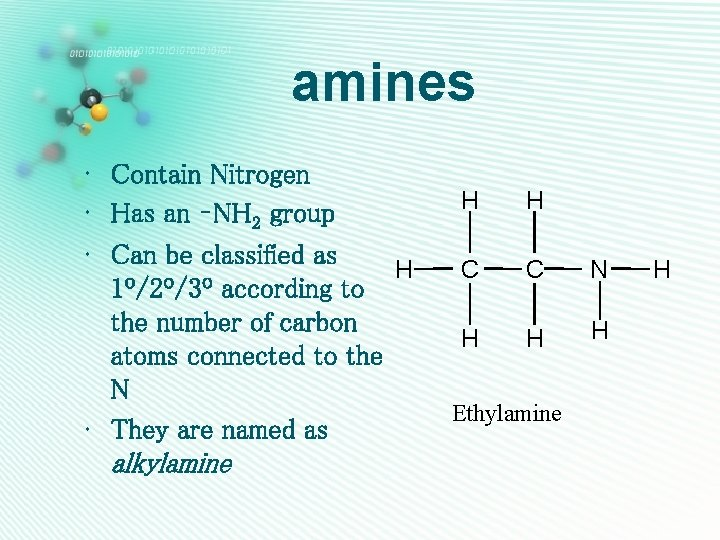amines • Contain Nitrogen • Has an –NH 2 group • Can be classified as H 1º/2º/3º according to the number of carbon atoms connected to the N • They are named as alkylamine H H C C N H H H Ethylamine H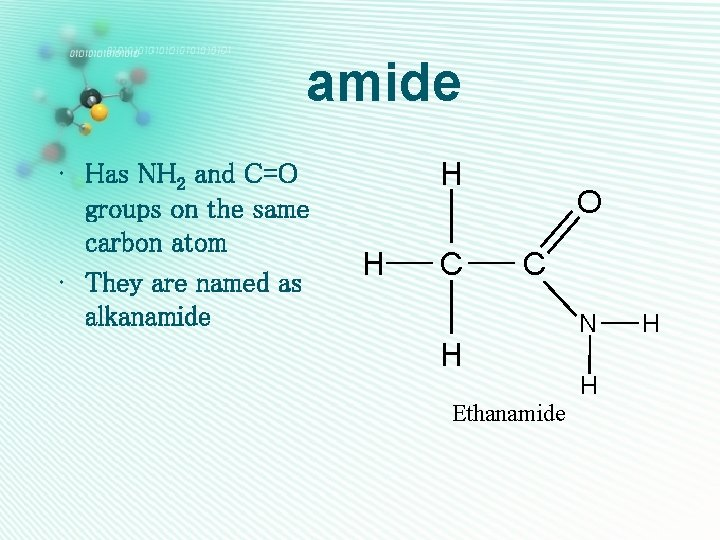amide • Has NH 2 and C=O groups on the same carbon atom • They are named as alkanamide H H C O C H Ethanamide N H H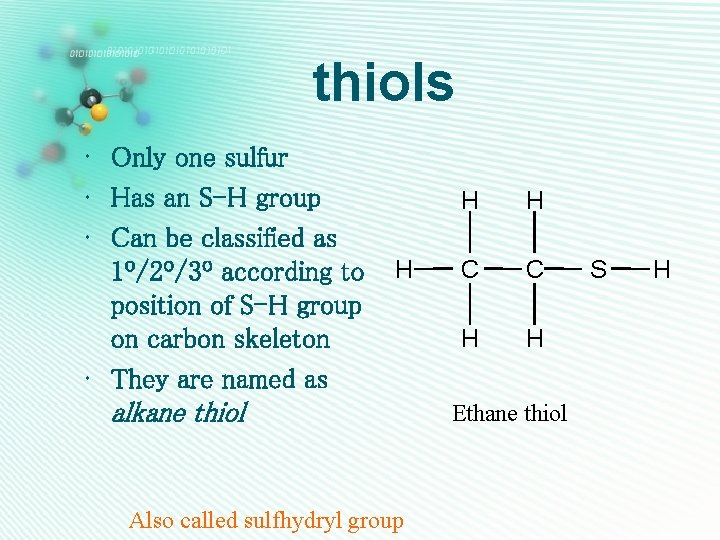thiols • Only one sulfur • Has an S-H group • Can be classified as 1º/2º/3º according to position of S-H group on carbon skeleton • They are named as alkane thiol H Also called sulfhydryl group H H C C H H Ethane thiol S H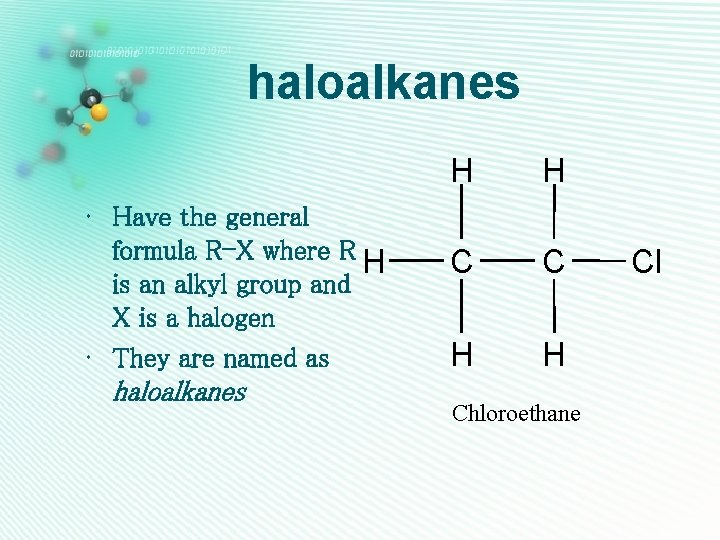haloalkanes • Have the general formula R-X where R H is an alkyl group and X is a halogen • They are named as haloalkanes H H C C H H Chloroethane Cl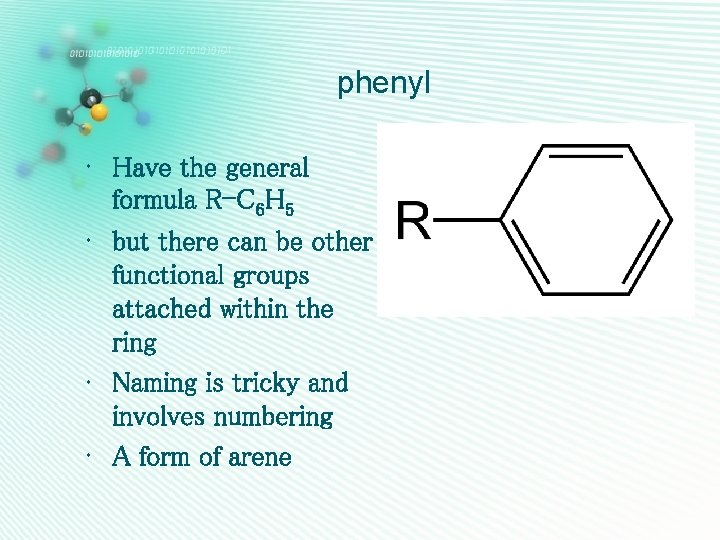phenyl • Have the general formula R-C 6 H 5 • but there can be other functional groups attached within the ring • Naming is tricky and involves numbering • A form of arene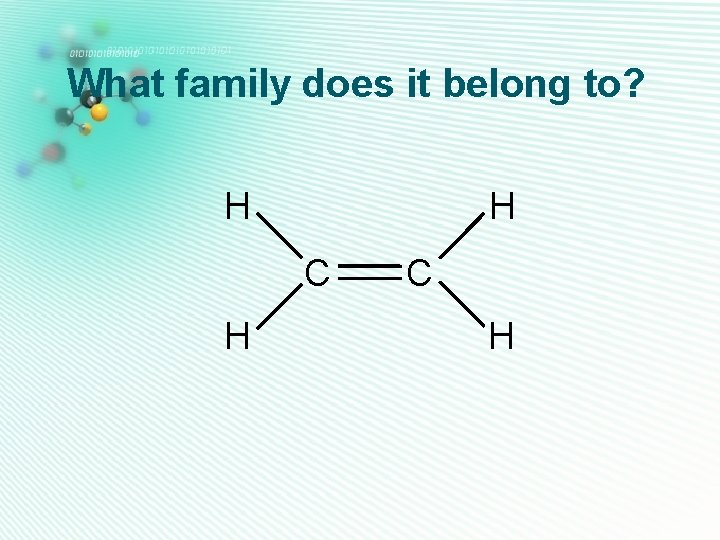What family does it belong to? H H C H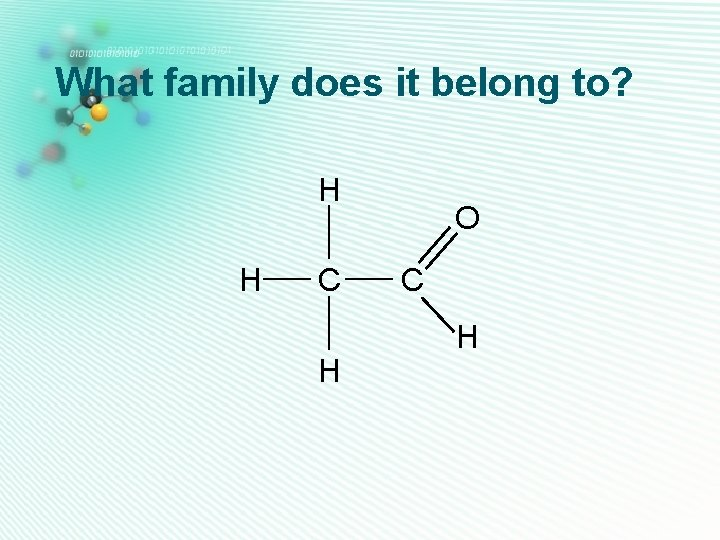What family does it belong to? H H C H O C HWhat family does it belong to? H H H O H C C C H HWhat family does it belong to? H H C H H O C H H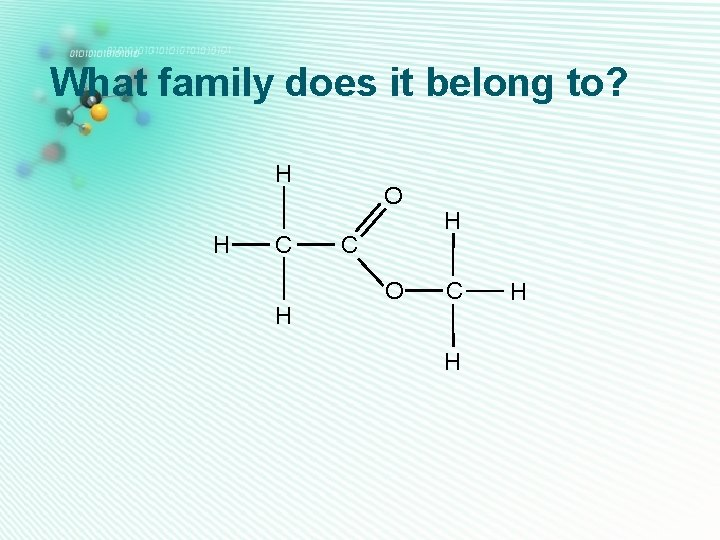What family does it belong to? H H C H O C O H C H H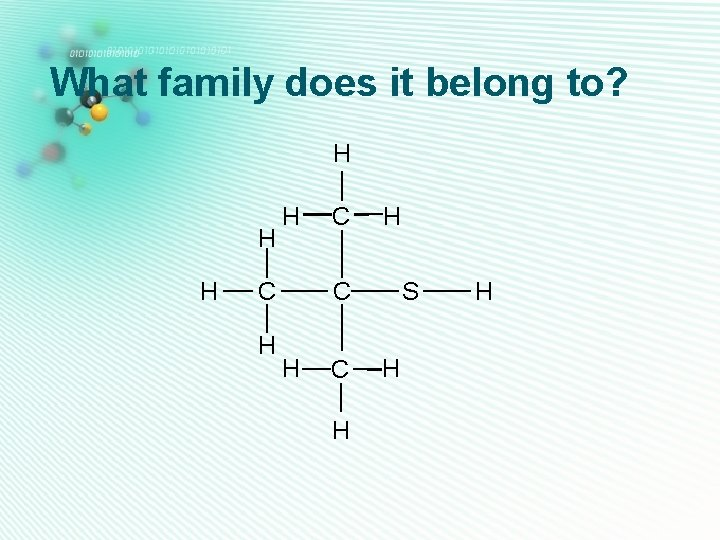What family does it belong to? H H H C C H H C S H H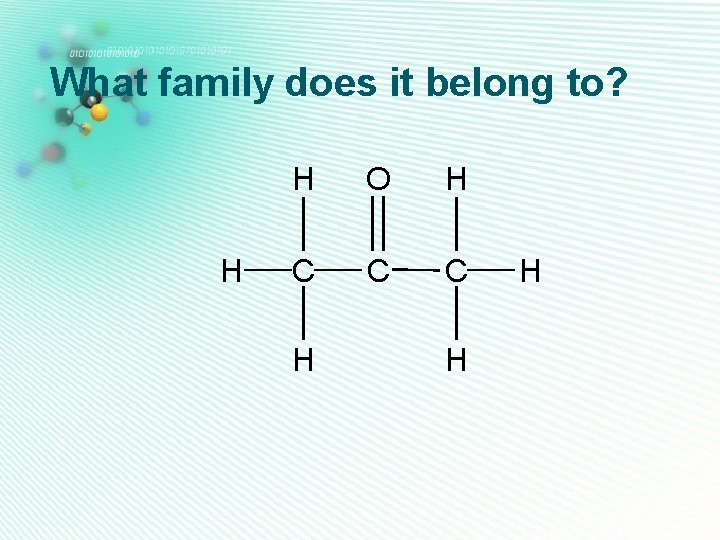What family does it belong to? H H O H C C C H H H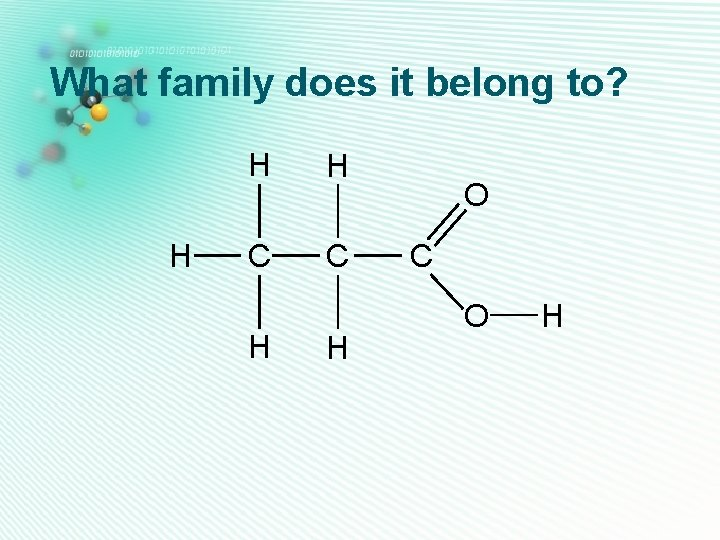What family does it belong to? H H H C C H H O C O H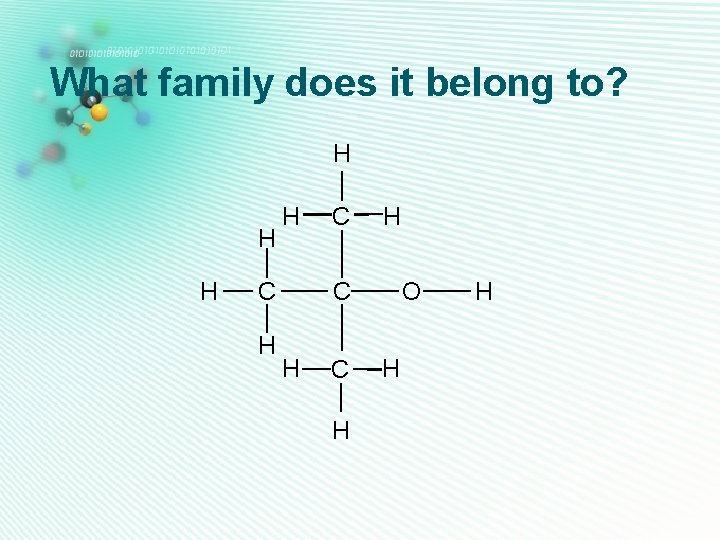What family does it belong to? H H H C C H H C O H HPredict Water Solubility As an in-class activity, we will predict molecules’ solubility based on the functional groups present and the # of carbon atoms. Hydrogen bonding increases water solubility.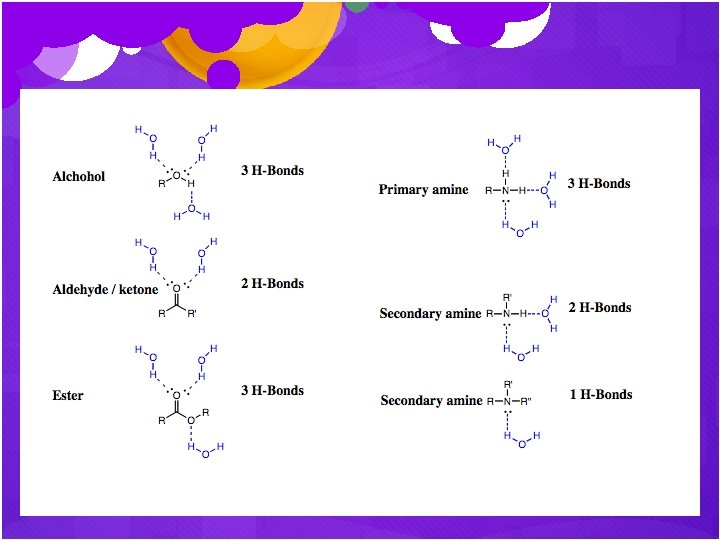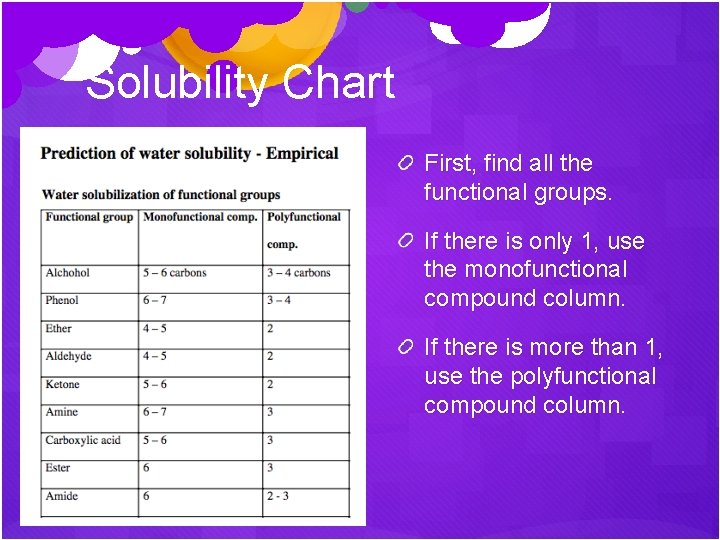Solubility Chart First, find all the functional groups. If there is only 1, use the monofunctional compound column. If there is more than 1, use the polyfunctional compound column.Solubility Chart The number there tells you the maximum # of carbons that can be in that molecule for it to be water soluble. If there is more than 1 functional group, add up the numbers. (Note: phenol is any benzene ring of C 6 H 5 bonded to an -OH)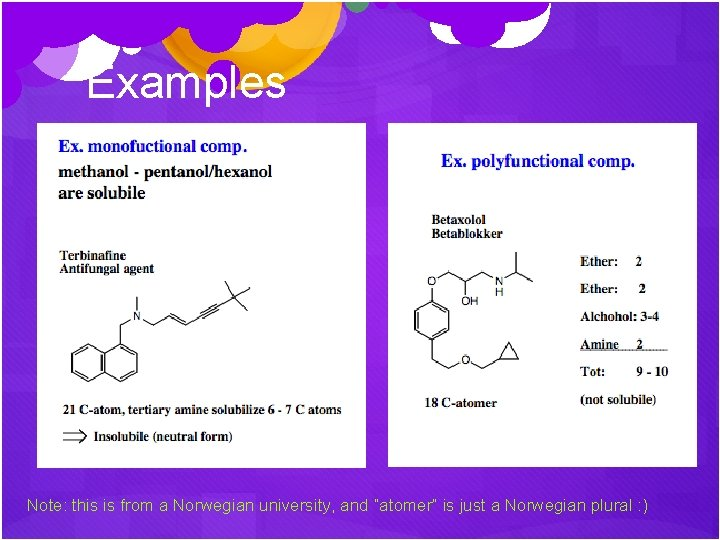Examples Note: this is from a Norwegian university, and “atomer” is just a Norwegian plural : )